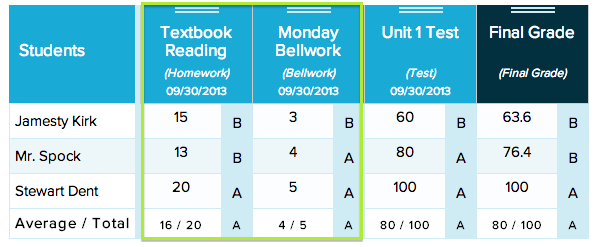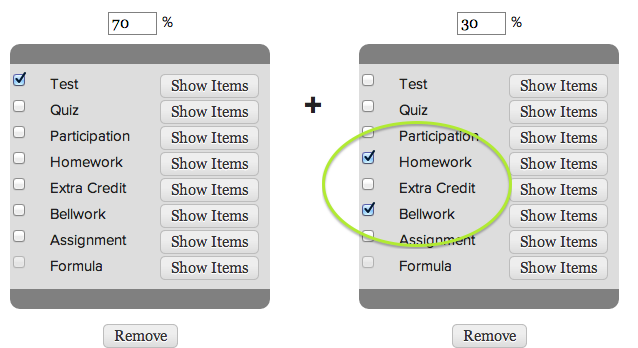# Weighted Formulas in Gradebook (Part 2)

This is yet another guest post by our chat agent – Anna

As awesome as automatically generated weighted final grades are in the Gradebook, there are many different features available with formulas, depending on how you would like to use it. Here are some other ideas for using formulas for weighted averages:

## Extra Credit

Want extra credit to be, say, a flat 10% of a student’s grade? To do this, add an extra weight factor for the category “extra credit” and set its weight percent at 10%. Of course, you can set extra credit to be any percent of the final grade that you choose.For example in the screenshot below, Stewart’s final grade is a 100%.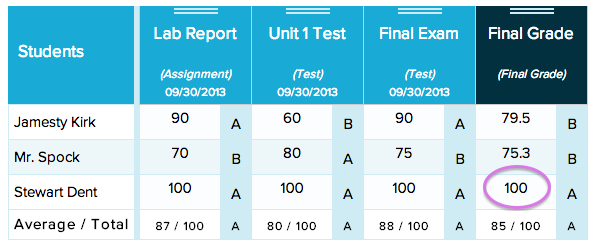In order to count extra credit, we use a formula like the one below that includes extra credit as 10% of the final grade.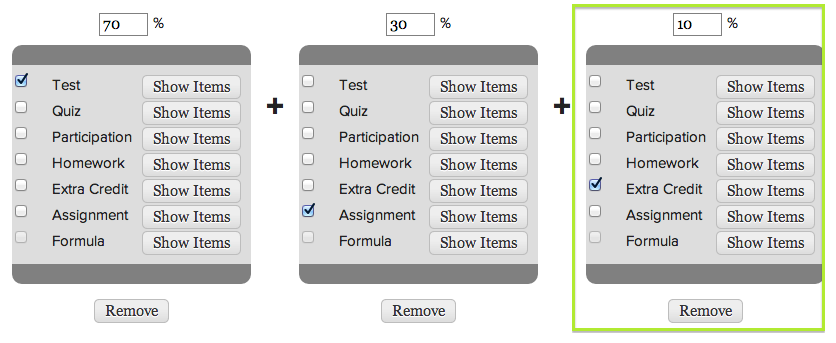Once the extra credit is taken into account, Stewart’s actual grade increases to 110%.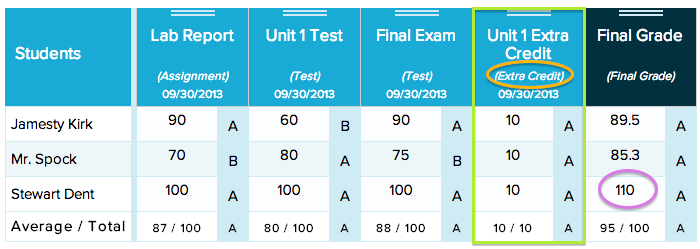By using weighted formula for weight percent-based extra credit, as students excel in class, their grade can be scaled up to reflect the extra credit they have earned.

Additional formulas are helpful for times when you might want to average just a few categories together. For example, if you you are looking for an “assessments” average, which finds an average of exams, tests, and quizzes, you could use a weighted average that is not a final grade. For this, average, be sure to uncheck “is final grade?” The formula will appear in the gradebook for teachers, students, and parents, but it’s grade will not appear on a student’s Report Card.

## Single-Category Averages

You can also use formulas to average all of a student’s grades in one category. To do this, set the weight percent to 100% and only select the category you want to average.

## Combinations of Categories

The grades for a few different categories can combined so that a student’s grades on assignments from these categories are averaged and weighted together in the final grade. For example, if Bellwork and Homework together were supposed to be 20% of a student’s final grade, then you could use one weight factor for these two categories. Simply add a weight factor for 20% and check both categories Bellwork and Homework in the same box.